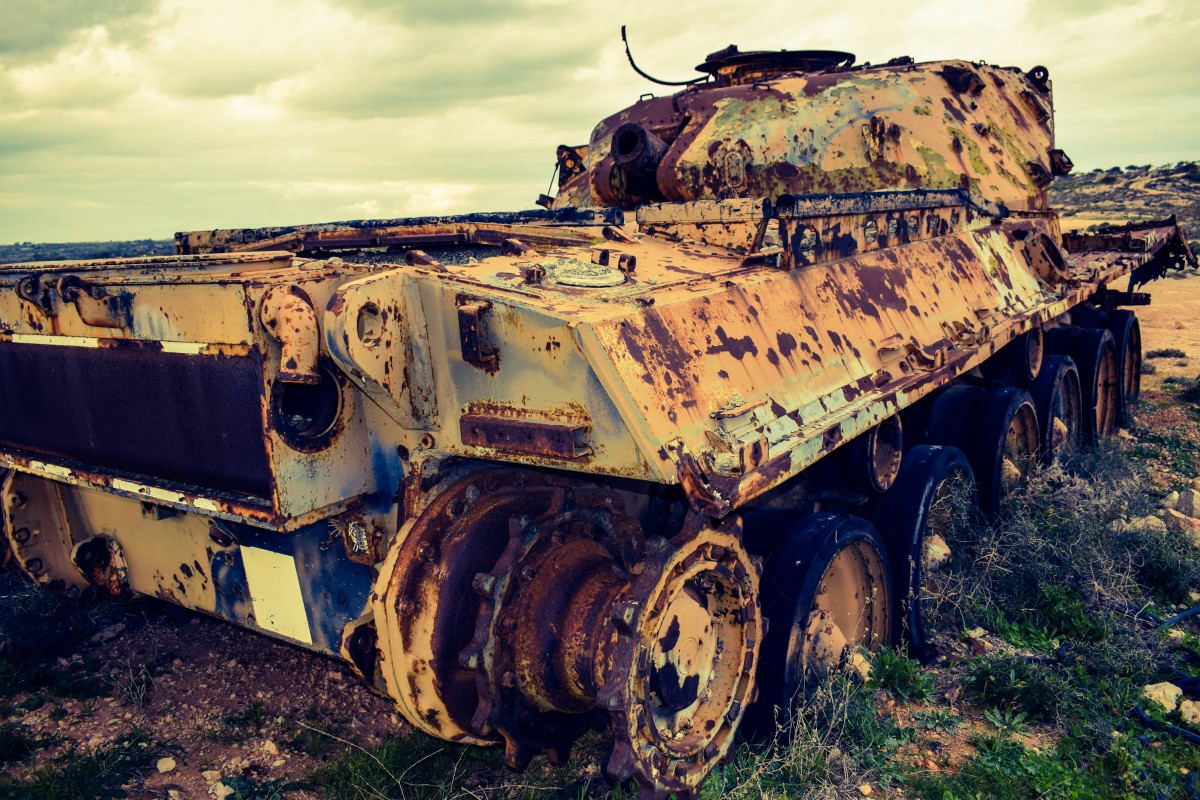> > Results on Depreciation

# Results on Depreciation

With the passage of time, every machinery faces wear and tear and therefore it’s value goes on decreasing. Even for any electronics goods or vehicle, it’s re-sell value is always less than the purchasing value. This is called depreciation. The value of all these goods decreases due to wear and tear, usage and many other factors.  Let us learn more about them.

### Suggested VideosArea of TrianglesCoin TossVST Permutations and Combinations Problem 1 and its Solution## Depreciation

The decrease in value is known as depreciation. Depreciation is nothing but an extension of a decrease in values.Source: Pixabay

And there is a formula which you can learn so that you can easily solve the questions related to the depreciation.
If the initial value of a product is I and rate of depreciation per year is at r % then final value F at the end of n years will be,

F = I × $$\frac{1 – r}{(100)^n}$$

Here are some of the questions based on the given formula.

### Examples

Q. The value of the laptop depreciates at the rate of 30 % per annum. The present value of the laptop if Rs. 40000. What will be the worth of laptop two years from now?

A. Rs. 28000
B. Rs  16000
C. Rs. 24400
D. Rs. 19600

Now we will solve this question by two methods. The first method is without any formula. This can help you when you don’t remember the depreciation formula. Method 1 will take more time and involves more calculation. In method 1, you can simply solve this question as the decrease in percentage.

The laptop depreciates annually by 30 %. So, there is a decrease of 30 % in the price of the laptop every year. For the 1st year, this change will be,
40000 x 30 x 1/100 = 12000 => 40000 – 12000 = Rs. 28000. So, for the second year, the base price of the laptop will be Rs. 28000. Now after reducing the price again by 30 %, the final price will be,
28000 x 30 x 1 / 100 = 8400 => 28000 – 8400 = Rs. 19600. So after 2 years, the final price of the laptop will be Rs. 19600.

Method 2

Here the initial value is given as Rs. 40000, the rate of interest is 30 % per annum, and the time period is 2 years. So, the final value,
F = 40000 x (1 – 70/100)²
=> 40000 x (0.30)²
=> 19600.
So, the correct answer is D.

### Depreciation in Months

Q. A car was bought at Rs. 12000. If the value of the car decreases by 1 % every year, what will be the value of the car after 3 months?

A. Rs. 11643
B. Rs. 11640
C. Rs. 11543
D. Rs. 11580

The initial value of the car is given as 12000. The rate of interest is at 1 % and the number of months is 3. In this question, you don’t need to convert months into years. So, the final formula will be,
F = 12000 x ( 1 – 1/100)³
=> 12000 x (0.99)³
=> 11643
So, the correct answer is A.

### Practice Questions

Q. The value of the scooter depreciates at the rate of 10 % per annum. The scooter was bought 3 years ago. What was the purchase price of the scooter if its current value is Rs. 8748?

A. Rs. 11000
B. Rs. 11500
C. Rs. 11800
D. Rs. 12000

Q. If the value of the car at the time of purchase was Rs. 4,00,000 and it depreciates at the rate of 6 % per annum for the next 4 years than what will the final value of the car after 4 years?

A. 312300
B. 313200
C. 313300
D. 313000

Q. The population in a town was 78000. It has been predicted that in next three the population will decrease at the rate of 5 %. What will be the population of town after 3 years?

A. 65650
B. 66875
C. 66785
D. 66700

Share with friends

## Customize your course in 30 seconds

##### Which class are you in?
5th
6th
7th
8th
9th
10th
11th
12th
Get ready for all-new Live Classes!
Now learn Live with India's best teachers. Join courses with the best schedule and enjoy fun and interactive classes.Ashhar Firdausi
IIT Roorkee
BiologyDr. Nazma Shaik
VTU
ChemistryGaurav Tiwari
APJAKTU
Physics
Get Started

## Browse

##### MensurationSubscribe
Notify of

## Question Mark?

Have a doubt at 3 am? Our experts are available 24x7. Connect with a tutor instantly and get your concepts cleared in less than 3 steps.